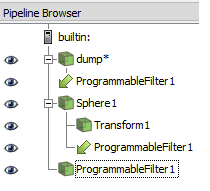# AttributeError: 'vtkCommonDataModelPython.vtkPolyData' object has no attribute 'SetCells'

Dear all,

I have the following problem. I am trying to plot ellipsoidal particles from a molecular dynamics run. The original data is divided in the following blocks (if paraview LAMMPS dump file reader used) the dimensions of the simulation box, and arrays containing particle id, particle type; x, y ,z coordinates; ; ;3 shape parameters; and 4 quaternions to mark the orientation of the particles.

When using a custom reader this data is transformed and quaternions automatically converted to rotation matrices. After I read my dump fileseries, I create a sphere source and attach a transform filter to it. Also I create a programable filter that points to both the dump data and the sphere source. My data pipeline looks like this.The code for the programable filter is the followin:

``````input1 = self.GetInputDataObject(0, 0) #threshold
input2 = self.GetInputDataObject(0, 1) #body
output = self.GetOutputDataObject(0)

newPoints = vtk.vtkPoints()
newcells = vtk.vtkCellArray()

p = input1.GetPointData()
B = input2.GetPointData()

BODIES=input1.GetNumberOfPoints()
EDGES=input2.GetNumberOfPoints()
SURFACES=input2.GetNumberOfCells()
c = 0
for body in range(0,BODIES):
r=input1.GetPoint(body)
M=p.GetAbstractArray('M')
T11=M.GetComponent(body,0)
T12=M.GetComponent(body,1)
T13=M.GetComponent(body,2)
T21=M.GetComponent(body,3)
T22=M.GetComponent(body,4)
T23=M.GetComponent(body,5)
T31=M.GetComponent(body,6)
T32=M.GetComponent(body,7)
T33=M.GetComponent(body,8)
for surface in range(0,SURFACES):
cell = input2.GetCell(surface)
COUNT=cell.GetNumberOfPoints()
for j in range(0,COUNT):
id=cell.GetPointId(j)
coord = input2.GetPoint(id)
x, y, z = coord[:3]
xnew=T11*x+T12*y+T13*z+r
ynew=T21*x+T22*y+T23*z+r
znew=T31*x+T32*y+T33*z+r
newPoints.InsertPoint(c, xnew, ynew, znew)
c += 1
newcells.InsertNextCell(3)
newcells.InsertCellPoint(c-1)
newcells.InsertCellPoint(c-2)
newcells.InsertCellPoint(c-3)

output.SetPoints(newPoints)
output.SetCells(5,newcells)
``````

However, when I run this code I find the following error ‘AttributeError: ‘vtkCommonDataModelPython.vtkPolyData’ object has no attribute ‘SetCells’’

Additionally I would like to find a way of additionally scaling the ellipsoids. This is, reading the particle shapes and transforming not only the orientation but also the size of the ellipsoids. But it is not clear to me how to do the position of the cells and I was wondering if somebody has some experience in this.

I believe ‘vtkCommonDataModelPython.vtkPolyData’ does not have cell attributes, you must change the output type of the programmable filter to ‘vtkUnstructuredGrid’ which has a SetCells function.